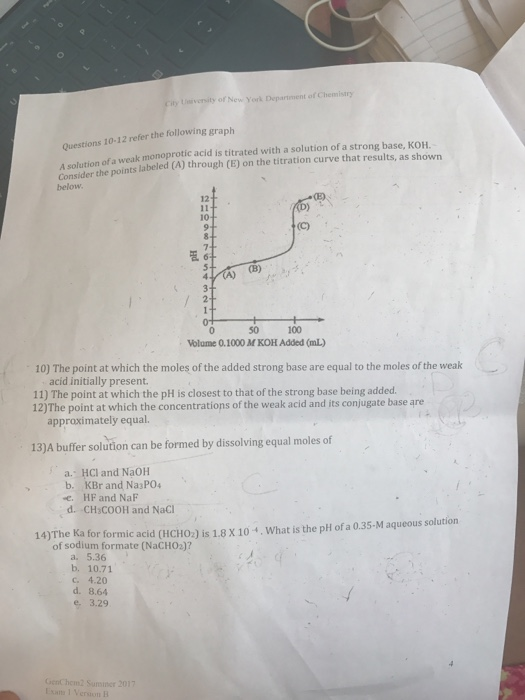# Question & Answer: Ueiversity of New York Department of Chemistry Questions 10-12 refer the following graph n…..Ueiversity of New York Department of Chemistry Questions 10-12 refer the following graph n of a weak monoprotic acid is titrated with a solution of a strong base, KOH. A solution of a Consider the points labeled (A) through (E) on the titration curve that results, as shown 12 10 (B) ),기 50 Volume 0.1000 M KOH Added (mL) 100 10) The point at which the moles of the added strong base are equal to the moles of the weak acid initially present. 11) The point at which the pH is closest to that of the strong base being added 12)The point at which the concentrations of the weak acid and jts conjugate base are approximately equal. 13)A buffer solution can be formed by dissolving equal moles of a. HCl and NaOH b. KBr and Na3P04 , HF and NaF d. CH3COOH and NaCl 14)The Ka for formic acid (HCHO2) is 1.8 x 10 , What is the pH of a 0.35-M aqueous solution of sodium formate (NaCH02)? a. 5.36 b. 10.71 c. 4.20 d. 8.64 e 3.29 GenChem2 Suminer 2017